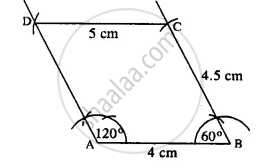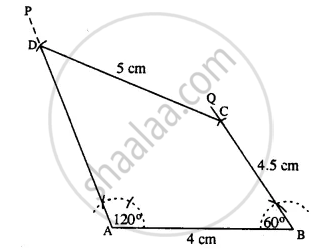# Construct a Quadrilateral Abcd in Which ; ∠A = 120°, ∠B = 60°, Ab = 4 Cm, Bc = 4.5 Cm and Cd = 5 Cm. - Mathematics

Sum

Construct a quadrilateral ABCD in which ; ∠A = 120°, ∠B = 60°, AB = 4 cm, BC = 4.5 cm and CD = 5 cm.

#### Solution

The rough figure is as follow :The Actual figure is constructed as followSteps :

1. Draw AB = 4 cm.
2. At A, draw ∠PAB = 120°.
3. At B, draw ∠QBA = 60°.
4. From BQ, cut BC = 4.5 cm.
5. From C, draw an arc of radius 5 cm which meets AP at D.
6. Join CD.
Thus ABCD is the required quadrilateral.
Is there an error in this question or solution?

#### APPEARS IN

Selina Concise Mathematics Class 8 ICSE
Chapter 18 Constructions
Exercise 18 (D) | Q 6 | Page 212